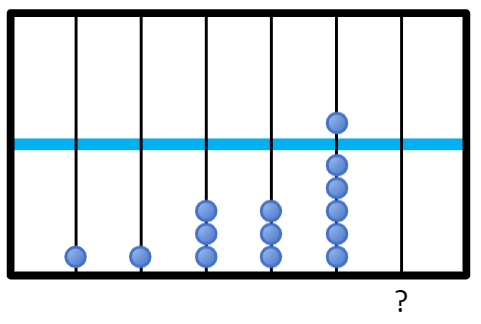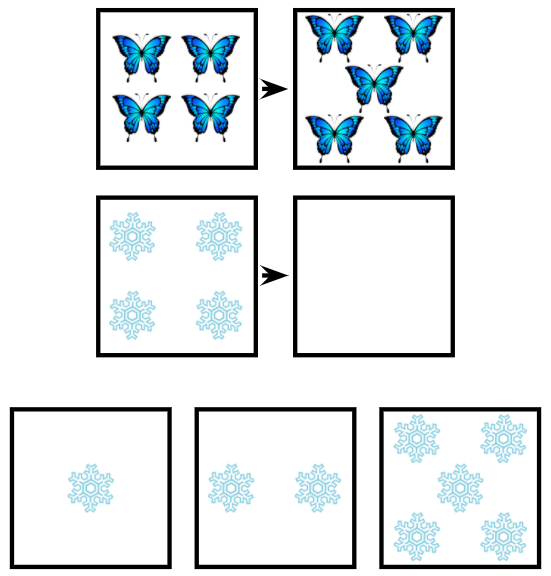# CogAT Quantitative Battery: Question Types & Sample Questions

The Quantitative Battery is one of three sections found on the CogAT. Learn about the question types found in this section, try some free sample questions, and learn how Elm Academy can help your child be prepared for test day.## What is the CogAT Quantitative Battery?

The CogAT, or Cognitive Abilities Test, is a standardized multiple-choice test frequently used to assess students for gifted and talented programs. The test is contains three sections, called batteries: the Nonverbal Battery, the Verbal Battery, and the Quantitative Battery.

The Quantitative Battery is designed to assess a child’s numerical reasoning skills, as well as their ability to detect patterns with numbers. For grades K-2 (Levels 5/6-8), questions are all entirely picture-based, with questions containing only images and figures. For grades 3 and up (Levels 9-17/18), questions have a more traditional numerical format.

The Quantitative Battery contains three unique question types: Number Analogies, Number Puzzles, and Number Series. Let’s take a look at each one in more detail below.

### Number Analogies

Number Analogies are basic comparison questions. You will be shown a pair, or multiple pairs, of numbers, and then have to figure out what the missing number is that completes the last pair. For grades K-2, the analogies will be in picture format, and your child will need to count the number of objects in each row. For grades 3 and up, the analogies will be written out as numbers.

Quick TipThe relationship between a pair of numbers must work for every pair. If it doesn’t work for every pair with the answer choice you pick, then it is not the correct answer.

### Number Analogies Sample Questions

#### Number Analogies - Level 5/6-8Answer: The correct answer is the 5 snowflakes. In the top row, the first box has 4 butterflies. The second box has 5 butterflies, meaning that 1 butterfly was added.

The bottom row should follow the same pattern. In the first box, there are 4 snowflakes. If we add 1 snowflake, this will give us 5 snowflakes as the correct answer.

#### Number Analogies - Level 9-17/18

[4 → 28]      [7 → 49]      [9 → ?]

a. 63

b. 56

c. 54

d. 72

e. 62

Answer: The correct answer is 63. The first pair of numbers is 4 and 28. The relationship is that 28 is 4 multiplied by 7 (4 x 7 = 28). The second pair of numbers is 7 and 49, and we have the same relationship here: 49 is 7 multiplied by 7 (7 x 7 = 49). The last pair of numbers must also follow the same pattern, meaning that the missing number will be 9 multiplied by 7, giving us 63 (9 x 7 = 63).

For detailed Number Analogies lessons and practice questions, check out our full CogAT courses.

### Number Puzzles

Number Puzzles questions are basic arithmetic questions, with a twist: these are more like algebra questions that require you to solve for a variable. For grades K-2, these questions will be in picture format, with trains carrying a certain number of objects on either side of the equation. For grades 3 and up, questions have a more traditional numerical format.

Quick Tip: Remember that both sides of the equation must always be equal to each other.

### Number Puzzles Sample Questions

#### Number Puzzles - Level 5/6-8Answer: The correct answer is 2 tennis balls. In the first train, there are 4 tennis balls. In the second train, there are 2 tennis balls. We need to find how many tennis balls go in the last train car to make the two sides equal. If we add 2 tennis balls to 2 tennis balls, we get 4 tennis balls.

#### Number Puzzles - Level 9-17/18

71 = 34 + 16 + ?

a. 20

b. 21

c. 53

d. 19

e. 31

Answer: The correct answer is 21. To solve this question, we need to first be aware that the numbers on either side of the equals sign must be the same. This means that 34 + 16 + ? must add up to 71. Next, we can add 34 and 16 together to get 50, so that we have:

71 = 50 + ?

Now we just need to get the ? by itself, so we subtract 50 from both sides. This gives us:

71 – 50 = 50 + ? – 50

This then simplifies to give us the final answer:

21 = ?

For detailed Number Puzzles lessons and practice questions, check out our full CogAT courses.

### Number Series

Number Series questions will show you a series of numbers, and you will have to figure out what number comes next in the sequence. In order to determine the next number, you will first need to figure out the pattern that exists in the series. For grades K-2, questions will use an abacus with specific numbers of beads to portray the sequence. Grades 3 and up use a more traditional numerical format.

Quick Tip: Start off by seeing if you can find a pattern between the first and second numbers, and then if that same pattern exists between the second and third numbers. If it doesn’t, that means there may be more than one pattern in the series.

### Number Series Sample Questions

#### Number Series - Level 5/6-8Answer: The correct answer is 8 beads. From left to right, there are 2, 4, and 6 beads. This means that in each row, the number of beads increases by 2. If we continue this pattern, the next row will have 2 more than 6, which is 8 beads.

#### Number Series - Level 9-17/18

5    12    9    16    13    ?

a. 10

b. 19

c. 20

d. 6

e. 16

Answer: The correct answer is 20. The first number in the series is 5. The next number is 12, meaning that 7 was added. The next number is 9, meaning that 3 was subtracted. The next number is 16, meaning that 7 was added again. This means the pattern in this series is +7, – 3. Following this pattern, we should add 7 to 13, giving us 20 as the correct answer.

For detailed Number Series lessons and practice questions, check out our full CogAT courses.

## Free CogAT Practice

Try out our free sample courses, which include lesson excerpts from our full courses, and a short CogAT quiz that includes one question from each specific question type found on the CogAT.

## CogAT Practice Tests & Preparation

For a more comprehensive practice experience, check out our full CogAT courses, which include a parent guide, detailed lessons for each question type found on the CogAT, 9 quizzes, and a full-length CogAT practice test.Daniela B
Great experience! My son who is 10 y.o. and gifted in math, enjoyed practicing all tests (verbal, quantitative, and non verbal). He had fun going through the lessons and quizzes (especially the long quizzes at the end of each category) over one weekend prior to taking the real CogAT tests at his school the very next week. He was well prepared and very excited to take the real tests. He was the only one who actually finished the real CogAT nonverbal test (the hardest, in my opinion) in his testing group at school. I loved how the lessons were structured and easy to follow. I highly recommend this program. Being prepared makes a big difference even for highly gifted children as my son.Elizabeth Gleinser